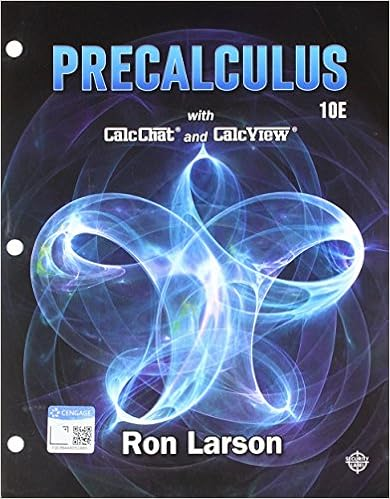Test3Review - MA 114-001 Fall 2014 Test 3 Review Note that...

• Notes
• 4
• 50% (2) 1 out of 2 people found this document helpful

This preview shows page 1 - 2 out of 4 pages.

We have textbook solutions for you!
The document you are viewing contains questions related to this textbook.The document you are viewing contains questions related to this textbook.
Chapter 9 / Exercise 17
Precalculus
Hostetler/LarsonExpert Verified
MA 114-001, Fall 2014 — Test 3 Review Note that all work must be shown (or explained, as the case may be) to guarantee full credit. Also, keep all numbers as fractions when necessary (i.e. when dividing 1 by 3, 2 by 7, etc.) and simplify fractions as much as possible. 1. ( Points) For each given set, draw a two-circle Venn diagram and shade the portion corresponding to it. (a) ( S 0 \ T ) [ ( S \ T ) (b) ( S \ T 0 ) [ ( S 0 [ T ) (c) S 0 \ T 0 2. ( Points) For each given set, draw a three-circle Venn diagram and shade the portion corre- sponding to it. (a) ( R \ S \ T ) 0 (b) R \ ( S [ T ) (c) ( R \ T 0 ) [ ( S \ T ) 3. ( Points) A merchant surveed 400 people to determine from what source they found out about a sale. The results of the survey are: 180 from the Internet 190 from television 190 from newspapers 80 from the Internet and television 90 from the Internet and newspapers 50 from television and newspapers 30 from all sources (a) Draw a Venn diagram that represents the given data and determine the number in each basic region. (b) How many people learned of the sale from newspapers or the Internet? (c) How many people learned of the sale only from newspapers? (d) How many people learned of the sale from the Internet or television but not newspapers? (e) How many people learned of the sale from at least two of the three media? (f) How many people learned of the sale from exactly one of the three media? 4. ( Points) Let R , S , and T be subsets of the universal set U . Let n ( U ) = 64, n ( R [ S [ T ) = 45, n ( R ) = 22, n ( T ) = 26, n ( R \ S ) = 4, n ( S \ T ) = 6, n ( R \ T ) = 8, and n ( R \ S \ T ) = 1. Draw an appropriate Venn diagram and use the given data to determine the number fo elements in each basic region. 5. ( Points) A particular bridge association has 3200 sanctioned clubs. Each club holds 2 games
We have textbook solutions for you!
The document you are viewing contains questions related to this textbook.The document you are viewing contains questions related to this textbook.
Chapter 9 / Exercise 17
Precalculus
Hostetler/LarsonExpert Verified
•••